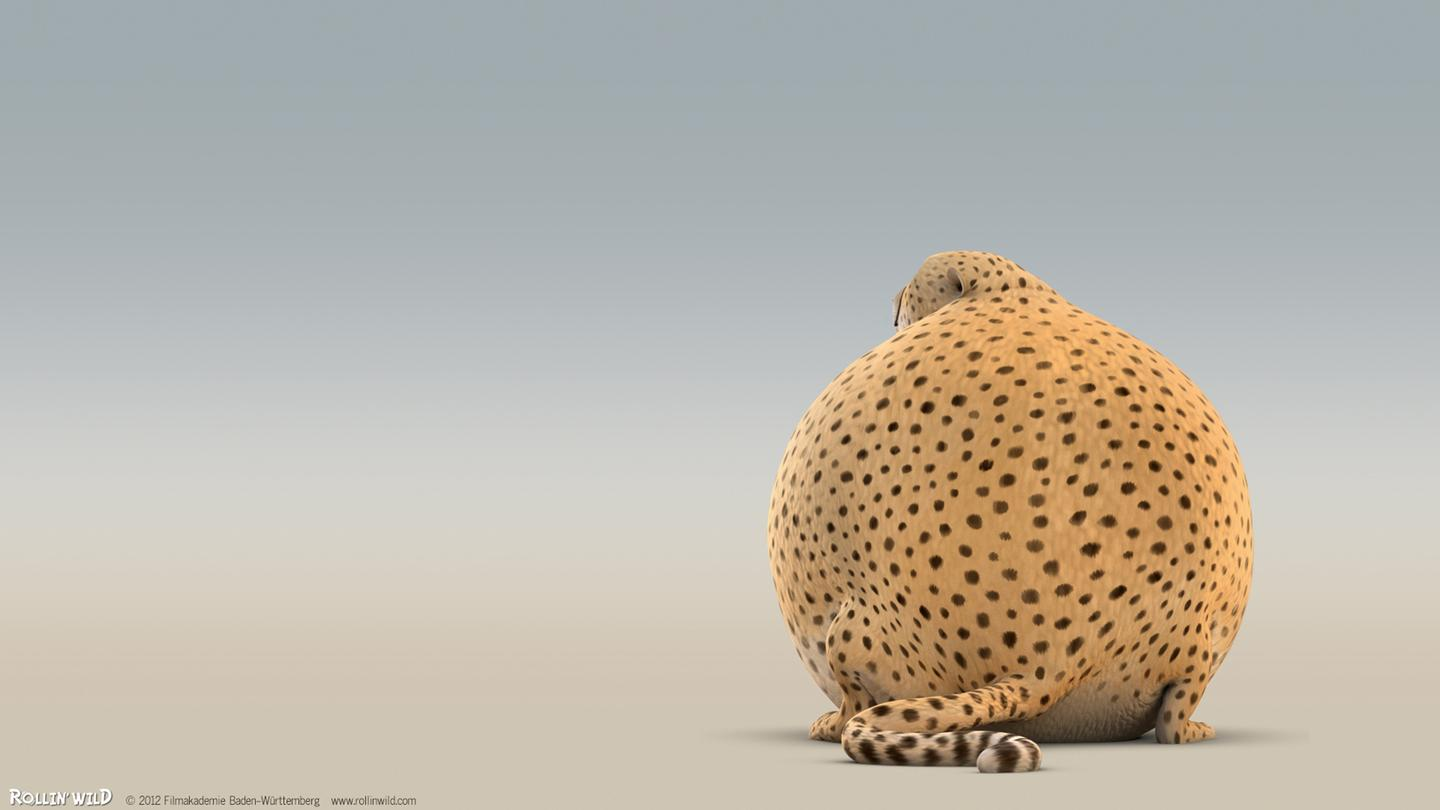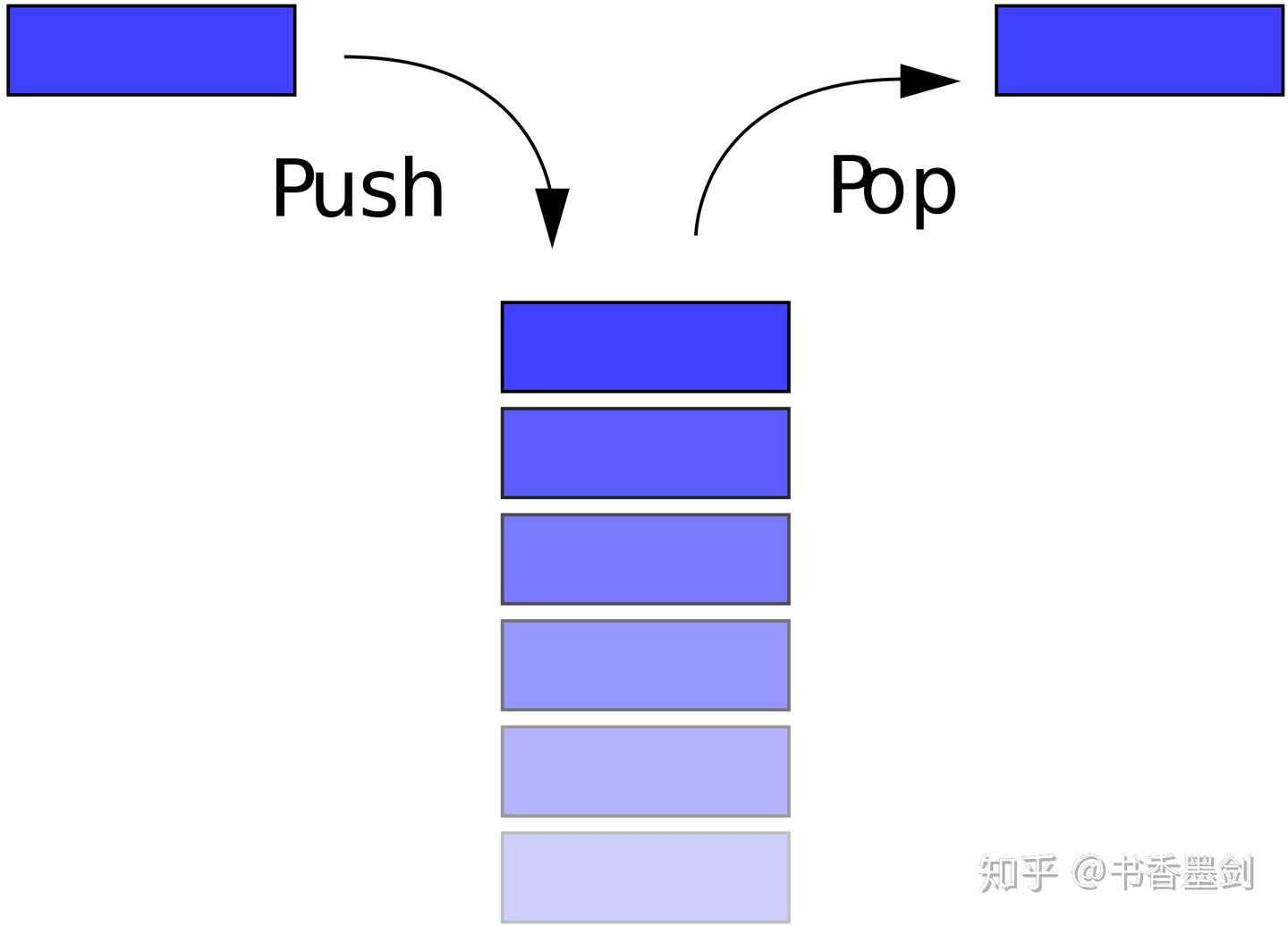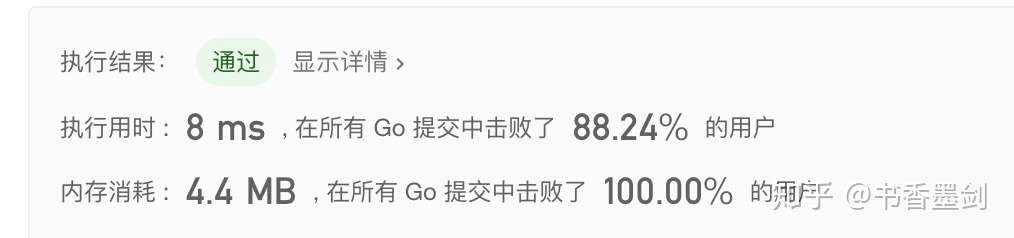# 【青铜三人行】每周一题之验证栈序列

## 验证栈序列

### 示例 1

``````输入：pushed = [1,2,3,4,5], popped = [4,5,3,2,1]

push(1), push(2), push(3), push(4), pop() -> 4,
push(5), pop() -> 5, pop() -> 3, pop() -> 2, pop() -> 1``````

### 示例 2

``````输入：pushed = [1,2,3,4,5], popped = [4,3,5,1,2]

### 提示

1. `0 <= pushed.length == popped.length <= 1000`
2. `0 <= pushed[i], popped[i] < 1000`
3. `pushed``popped` 的排列

## 栈结构

• push 操作，往栈顶放入一个数据。
• pop 操作，从栈顶取走一个数据。## 解题思路

``````function validateStackSequences(pushed, popped) {
const stack = [];

pushed.forEach(ele => {
stack.push(ele);
stack.pop();
});
return !stack.length;
}``````

• 按照 `pushed` 的顺序将元素 `push` 入栈。
• `push` 的过程中尝试 `pop` 元素。
• `pop` 元素的顺序要和 `poped` 的顺序一样。

``````function validateStackSequences(pushed, popped) {
const stack = [];
let popIndex = 0;

for (const val of pushed) {
stack.push(val);

while (stack.length !== 0 && stack[stack.length - 1] === popped[popIndex]) {
stack.pop();
popIndex++;
}
}
return stack.length === 0;
}``````

``````function validateStackSequences(pushed, popped) {
const stack = [];

pushed.forEach(ele => {
stack.push(ele);
while (stack.length && stack[stack.length - 1] === popped) {
stack.pop();
popped.shift();
}
});
return !stack.length;
}``````

### Extra Go

``````func validateStackSequences(pushed []int, popped []int) bool {

if len(popped) == 0 {
return true
}

pushedValues := make(map[int]int) //存储所有的已经入栈的值和数组索引

left := 0
right := 0

for i := 0; i < len(pushed); i++ {
if pushed[i] == popped {
pushed[i] = -1  // 出栈
left = i-1
right = i+1
break
} else {
pushedValues[pushed[i]] = i
}
}

for j := 1; j < len(popped); j++ {

if _, ok := pushedValues[popped[j]]; ok { // 值已经加入stack了
for left >= 0 {
if pushed[left] == -1 {
left--
} else {
break
}
}

if left < 0 {
left = 0
}

if popped[j] != pushed[left] {
return false
} else { // 值相等，出栈
pushed[left] = -1
left--
}
} else { // 值没有加入stack，继续往前找
for right < len(popped) {
if popped[j] == pushed[right] { // 找到了
pushed[right] = -1 //出栈
left = right - 1 // 重新赋值left
right ++  // 重新赋值right
break
} else { // 没有找到，继续往前
pushedValues[pushed[right]] = right
right++
}
}
}
}
return true
}``````